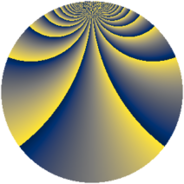# Properties

 Label 2850.2.ciLevel $2850$ Weight $2$ Character orbit 2850.ci Rep. character $\chi_{2850}(83,\cdot)$ Character field $\Q(\zeta_{60})$ Dimension $3200$ Sturm bound $1200$

# Learn more about

## Defining parameters

 Level: $$N$$ $$=$$ $$2850 = 2 \cdot 3 \cdot 5^{2} \cdot 19$$ Weight: $$k$$ $$=$$ $$2$$ Character orbit: $$[\chi]$$ $$=$$ 2850.ci (of order $$60$$ and degree $$16$$) Character conductor: $$\operatorname{cond}(\chi)$$ $$=$$ $$1425$$ Character field: $$\Q(\zeta_{60})$$ Sturm bound: $$1200$$

## Dimensions

The following table gives the dimensions of various subspaces of $$M_{2}(2850, [\chi])$$.

Total New Old
Modular forms 9728 3200 6528
Cusp forms 9472 3200 6272
Eisenstein series 256 0 256

## Trace form

 $$3200q + O(q^{10})$$ $$3200q + 16q^{13} - 12q^{15} - 400q^{16} - 32q^{18} + 40q^{19} - 32q^{22} - 8q^{25} - 24q^{27} - 12q^{33} + 16q^{37} + 80q^{39} + 32q^{42} + 40q^{43} + 216q^{45} - 16q^{52} + 8q^{55} - 68q^{57} + 8q^{60} + 52q^{63} - 136q^{67} + 8q^{70} - 16q^{72} - 64q^{73} - 296q^{75} - 16q^{78} + 240q^{84} + 48q^{85} + 32q^{87} - 16q^{88} + 4q^{90} + 20q^{93} + 16q^{97} + O(q^{100})$$

## Decomposition of $$S_{2}^{\mathrm{new}}(2850, [\chi])$$ into newform subspaces

The newforms in this space have not yet been added to the LMFDB.

## Decomposition of $$S_{2}^{\mathrm{old}}(2850, [\chi])$$ into lower level spaces

$$S_{2}^{\mathrm{old}}(2850, [\chi]) \cong$$ $$S_{2}^{\mathrm{new}}(1425, [\chi])$$$$^{\oplus 2}$$